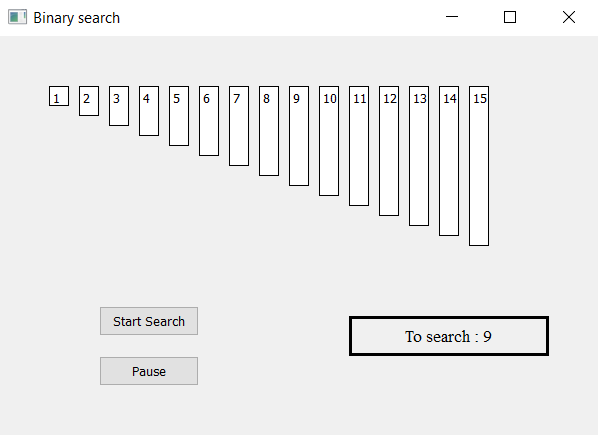Related Articles

# PyQt5 – Binary Search Visualizer

• Last Updated : 03 May, 2020

In this article we will see how we can make a PyQt5 application which will visualize the Binary search algorithm.

Binary Search: Search a sorted array by repeatedly dividing the search interval in half. Begin with an interval covering the whole array. If the value of the search key is less than the item in the middle of the interval, narrow the interval to the lower half. Otherwise narrow it to the upper half. Repeatedly check until the value is found or the interval is empty.GUI implementation steps :
1. Create a list of label according to the given list of numbers
2. Set their text, border, color and geometry with respective gap from each other
3. Each label height should be proportional to the value of each number
4. Create a start and pause push button to start the searching and pause the searching
5. Create a result label to show the searching status

Algorithm implementation steps :
1. Create label list corresponding to the given numbers
2. Create variable for the first last and mid index and flag for searching.
3. Add action to the push button, their action should change the flag status i.e start action should make flag true and pause action should make flag false
4. Create timer object which calls a method after every specific time
5. Inside the timer method check for the flag is flag is true begin the binary search algorithm
6. Calculate mid value and check if mid value is equal then stop the search and make label green.
7. Show result as found else change the value of first and last search according to the mid value. Make the label grey color and continue the search.
8. If the index of first become greater then index of last stop the search and show result as not found.

Below is the implementation –

 `# importing libraries``from` `PyQt5.QtWidgets ``import` `*` `from` `PyQt5 ``import` `QtCore, QtGui``from` `PyQt5.QtGui ``import` `*` `from` `PyQt5.QtCore ``import` `*` `import` `sys`` ` ` ` `class` `Window(QMainWindow):`` ` `    ``# list of numbers``    ``number ``=` `[``1``, ``2``, ``3``, ``4``, ``5``, ``6``, ``7``, ``8``, ``9``, ``10``, ``11``, ``12``,  ``13``, ``14``, ``15``]`` ` `    ``def` `__init__(``self``):``        ``super``().__init__()`` ` `        ``# setting title``        ``self``.setWindowTitle(``"Binary search "``)`` ` `        ``# setting geometry``        ``self``.setGeometry(``100``, ``100``, ``600``, ``400``)`` ` `        ``# calling method``        ``self``.UiComponents()`` ` `        ``# showing all the widgets``        ``self``.show()`` ` `    ``# method for widgets``    ``def` `UiComponents(``self``):`` ` `        ``# start flag``        ``self``.start ``=` `False`` ` `        ``# list to hold labels``        ``self``.label_list ``=` `[]`` ` `        ``# desired value``        ``self``.desired ``=` `9`` ` `        ``# binary search variable``        ``self``.first ``=` `0``        ``self``.last ``=` `len``(``self``.number) ``-` `1``        ``self``.mid ``=` `0`` ` `        ``# local counter``        ``c ``=` `0`` ` `        ``# iterating list of numbers``        ``for` `i ``in` `self``.number:`` ` `            ``# creating label for each number``            ``label ``=` `QLabel(``str``(i), ``self``)`` ` `            ``# adding background color and border``            ``label.setStyleSheet("border : ``1px` `solid black; ``                                 ``background : white;")`` ` `            ``# aligning the text``            ``label.setAlignment(Qt.AlignTop)`` ` `            ``# setting geometry using local counter``            ``# first parameter is distance from left and ``            ``# second is distance from top``            ``# third is width and forth is height``            ``label.setGeometry(``50` `+` `c ``*` `30``, ``50``, ``20``, i ``*` `10` `+` `10``)`` ` `            ``# adding label to the label list``            ``self``.label_list.append(label)`` ` `            ``# incrementing local counter``            ``c ``=` `c ``+` `1`` ` ` ` `        ``# creating push button to start the search``        ``self``.search_button ``=` `QPushButton(``"Start Search"``, ``self``)`` ` `        ``# setting geometry of the button``        ``self``.search_button.setGeometry(``100``, ``270``, ``100``, ``30``)`` ` `        ``# adding action to the search button``        ``self``.search_button.clicked.connect(``self``.search_action)`` ` `        ``# creating push button to pause the search``        ``pause_button ``=` `QPushButton(``"Pause"``, ``self``)`` ` `        ``# setting geometry of the button``        ``pause_button.setGeometry(``100``, ``320``, ``100``, ``30``)`` ` `        ``# adding action to the search button``        ``pause_button.clicked.connect(``self``.pause_action)`` ` `        ``# creating label to show the result``        ``self``.result ``=` `QLabel(``"To search : "` `+` `str``(``self``.desired), ``self``)`` ` `        ``# setting geometry``        ``self``.result.setGeometry(``350``, ``280``, ``200``, ``40``)`` ` `        ``# setting style sheet``        ``self``.result.setStyleSheet(``"border : 3px solid black;"``)`` ` `        ``# adding font``        ``self``.result.setFont(QFont(``'Times'``, ``10``))`` ` `        ``# setting alignment``        ``self``.result.setAlignment(Qt.AlignCenter)`` ` `        ``# creating a timer object``        ``timer ``=` `QTimer(``self``)`` ` `        ``# adding action to timer``        ``timer.timeout.connect(``self``.showTime)`` ` `        ``# update the timer every 300 millisecond``        ``timer.start(``300``)`` ` `    ``# method called by timer``    ``def` `showTime(``self``):`` ` ` ` `        ``# checking if flag is true``        ``if` `self``.start:``            ``# implementing binary search``            ``# finding mid index``            ``self``.mid ``=` `(``self``.first ``+` `self``.last)``/``/``2`` ` `            ``# if first index become greater than last index``            ``if` `self``.first > ``self``.last:`` ` `                ``# make start flag false``                ``self``.start ``=` `False``                ``# show output as not found``                ``self``.result.setText(``"Not Found"``)`` ` `            ``# if mid value is equal to the desired value``            ``if` `self``.number[``self``.mid] ``=``=` `self``.desired:`` ` `                ``# make flag false``                ``self``.start ``=` `False`` ` `                ``# show output in result label``                ``self``.result.setText(``"Found at index : "` `+` `str``(``self``.mid))`` ` `                ``# set color of label to green``                ``self``.label_list[``self``.mid].setStyleSheet(``                              ``"border : 2px solid green; "``                              ``"background-color : lightgreen"``)`` ` `            ``# if not equal to mid value``            ``else``:``                ``# make color grey``                ``self``.label_list[``self``.mid].setStyleSheet(``                                   ``"border : 1px solid black; "``                                   ``"background-color : grey"``)`` ` `            ``# mid value is higher``            ``if` `self``.number[``self``.mid] > ``self``.desired:``                ``# change last index``                ``self``.last ``=` `self``.mid ``-` `1`` ` `            ``# if mid value is smaller``            ``if` `self``.number[``self``.mid] < ``self``.desired:``                ``# change first index``                ``self``.first ``=` `self``.mid ``+` `1`` ` ` ` ` ` `    ``# method called by search button``    ``def` `search_action(``self``):`` ` `        ``# making flag true``        ``self``.start ``=` `True`` ` `        ``# showing text in result label``        ``self``.result.setText(``"Started searching..."``)`` ` `    ``# method called by pause button``    ``def` `pause_action(``self``):`` ` `        ``# making flag false``        ``self``.start ``=` `False`` ` `        ``# showing text in result label``        ``self``.result.setText(``"Paused"``)`` ` ` ` `# create pyqt5 app``App ``=` `QApplication(sys.argv)`` ` `# create the instance of our Window``window ``=` `Window()`` ` `# start the app``sys.exit(App.``exec``())`

Output :

Attention geek! Strengthen your foundations with the Python Programming Foundation Course and learn the basics.

To begin with, your interview preparations Enhance your Data Structures concepts with the Python DS Course. And to begin with your Machine Learning Journey, join the Machine Learning – Basic Level Course

My Personal Notes arrow_drop_up